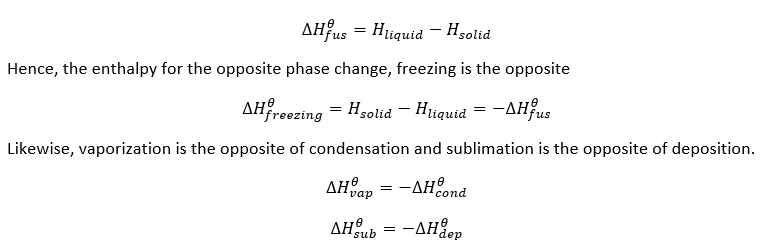# Phase transition in Thermodynamics

Chemical Thermodynamics: Phase transition

For any phase transition, enthalpy change can be experimentally quantified and tabulated. Each of the phase transitions has an associated latent heat which is required for the transformation.

The process that turns solid to liquid is referred to as fusion. The enthalpy of this change is calculated as:Figure : Phase transitions

The figure above presents the basic transitions that substances undergo due to changes in pressure and temperature. The processes in which energy is absorbed to weaken/break the intermolecular interactions (melting, vaporization and sublimation) are endothermic (so ΔH will be positive) in nature. Alternatively, processes in which energy is released to facilitate attractive interactions (freezing, condensation and deposition) are exothermic (so ΔH will be negative) in nature.

Molar enthalpy is the measure of attraction between particles of either solid for fusion or liquid for vaporization. In each case, 1 mole of a substance is analysed. Hence, enthalpy changes required to convert 1 mol of a solid to liquid atits melting point is called enthalpy of fusion. Enthalpy changes required to convert 1 mol of a liquid to a gas at its boiling point is called enthalpy of vaporization. Enthalpy changes required to convert 1 mol of solid to a gas at its triple point is called enthalpy of sublimation.

During melting (Solid –>Liquid) and evaporation (Liquid –> Gas), the energy is specifically used to transform the phase of a substance without any change of temperature. Therefore, melting and vaporization are isothermal processes. Melting and boiling of any substance are highly influenced by the surrounding pressure. Gases are more affected by the pressure than liquid so the change in pressure can cause a change in boiling point. Standard boiling point and melting point are hence denoted with the pressure of 1 atm. The same is valid for opposite phase transitions: freezing (liquid –> solid) and condensation (solid –> liquid).

During sublimation (Solid –> Gas) and deposition (Gas –> Solid) measurable energy goes into the conversion of phase. These phase transitions are also isothermal in nature. The relationship between sublimation and other enthalpies is shown below;

ΔHsub = ΔHdep = ΔHfus + ΔHvap

A heating curve is generally used to represent phase and state change. This graph clearly shows the relationship between enthalpy and phase change:Figure :Heating curve diagram

In the figure above, a solid substance gains kinetic energy resulting in temperature change. For the calculation of heat required for temperature change, specific heat (C) of solid phase is used. At the melting point, latent heat is absorbed to break intermolecular forces and increase kinetic energy to form liquid wherein the temperature remains constant. For the calculation of heat required for phase change, molar heat of fusion is used.The liquid then gains K.E to increase its temperature until its boiling point where specific heat of liquid is used for calculations. Liquid –> gas change requires latent heat for breaking the intermolecular forces between particles to form gas. Here molar heat of vaporization is required for calculations.

 Parameter Phase Transition Enthalpy molar heat of fusion (melting) solid –> liquid +6.0 kJ/mol molar heat of vaporization liquid –> gas +40.7 kJ/mol specific heat of ice Ice –> Ice 2.09 J/g•°C specific heat of the water Water –> Water 4.18 J/g•°C specific heat of steam Steam –> Steam 1.84 J/g•°C

Figure : Parameters used for transition of water from one state to another.